### Geometrical Constructions Class 9th Mathematics AP Board Solution

##### Question 1.Construct ∆ABC in which BC = 7 cm, ∠B = 75° and AB + AC = 12 m.Answer:Given: base BC = 7cm, AB + AC = 12 cm and∠B = 75° of Δ ABC.Required: To construct a ΔABCSteps of construction:Step 1: Draw a segment BC of length of 7 c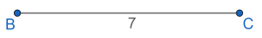Step 2: At vertex B, construct ∠B = 75° and produce a ray BP.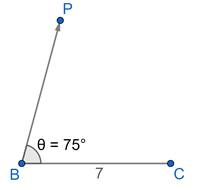Step 3: Mark an arc on ray BP cutting at D such that BD = 12cm.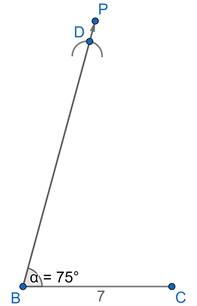Step 4: Draw segment CD.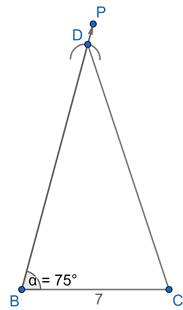Step 5: Construct the perpendicular bisector of segment CD.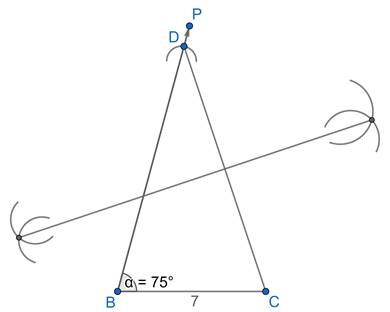Step 6: Name the point of intersection of ray BP and the perpendicular bisector of CD as A.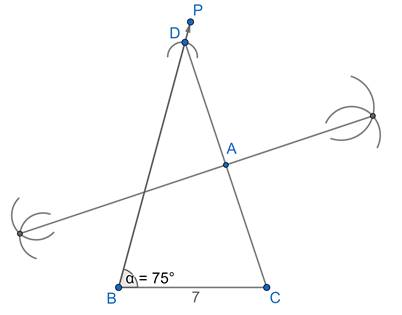Step 7: Draw segment AB.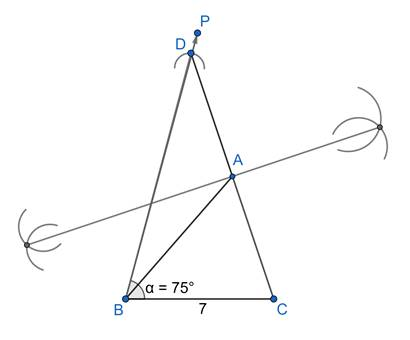ΔABC is the required triangle.Question 2.Construct PQR in which QR = 8 cm, ∠Q = 60° and PQ - PR = 3.5 cm.Answer:Given: Base QR = 8 cm, PQ-PR = 3.5cm and∠Q = 60° of Δ PQR.Required: To construct a triangle PQR.Steps of construction:Step 1: Draw a segment QR of length 8cm.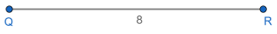Step 2: Draw ray QL such that ∠Q = 60°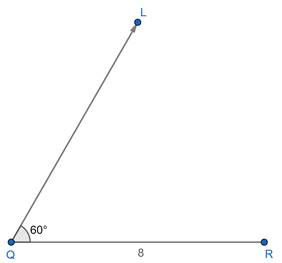Step 3: Mark an arc on opposite ray QL i.e. QS cutting at D such that QD = 3.5cm.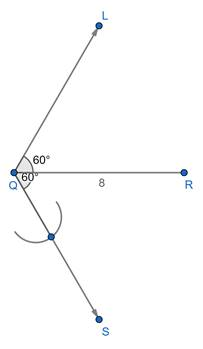Step 4: Draw segment RD.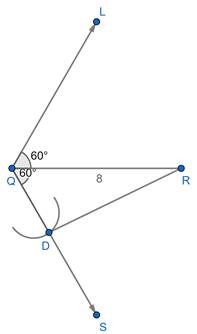Step 5: Construct the perpendicular bisector of segment RD.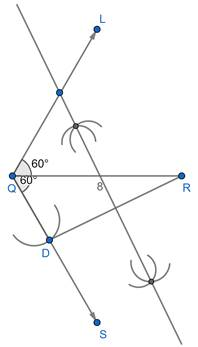Step 6: Name the point of intersection of ray QL and the perpendicular bisector of RD as P.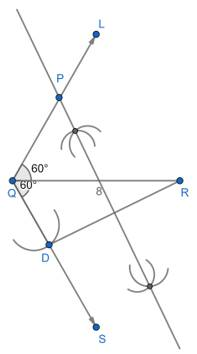Step 7: Draw segment PR.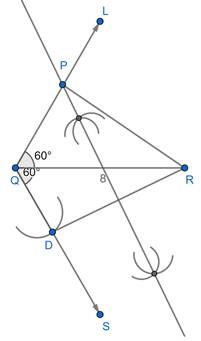ΔPQR is the required triangle.Question 3.Construct ∆XYZ in which ∠Y = 30°, ∠Z = 60° and XY + YZ + ZX = 10 cm.Answer:Given: ∠Y = 30°, ∠Z = 60° and perimeter of ΔXYZ = 10 cmSteps of construction:Step 1: Step 1: raw a line segment QR of 10.5cm.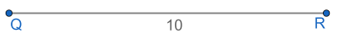Step 2: From point Q draw a ray QD at 30° and from R draw a ray RE at 60°.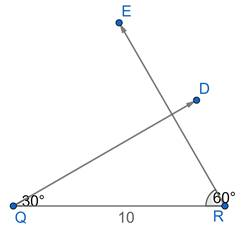Step 3: Draw an angle bisector of Q and R, two angle bisectors intersect each other at point X.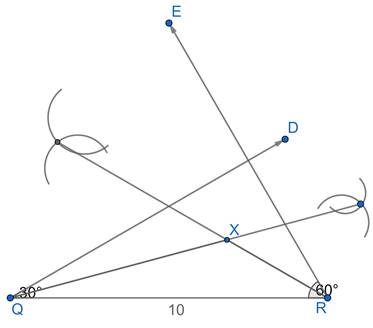Step 4: Draw a line bisector of QX and XR respectively these two-line bisectors intersect at point Y and Z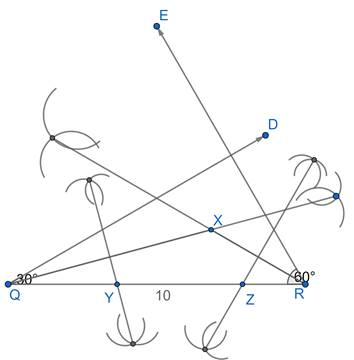Step 5: Join XY AND XZ.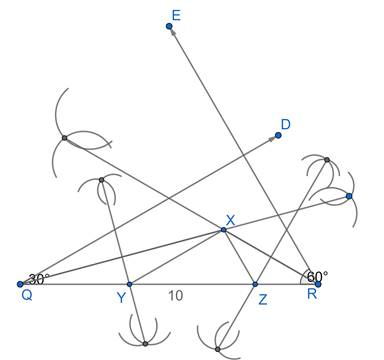Step 6: Δ XYZ is required triangle.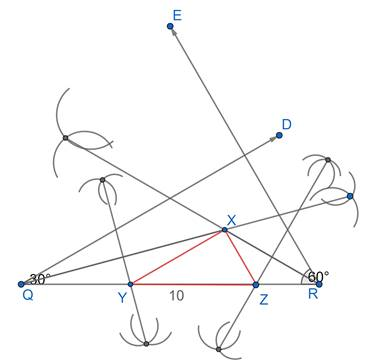Question 4.Construct a right triangle whose base is 7.5cm. and sum of its hypotenuse and other side is 15cm.Answer:Given base(BC) = 7.5cm and AB + AC = 15cm and ∠B = 90°Steps of construction:Step 1: Draw the base BC = 7.5cm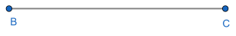Step 2: Make an ∠XBC = 90° at the point B of base BC.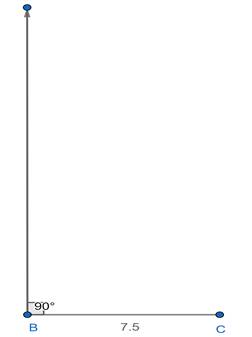Step 3: Cut the line segment BD equals to AB + AC i.e. 15cm from the rayXB.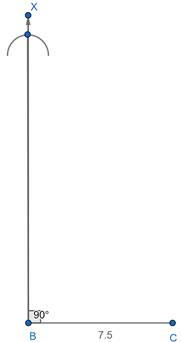Step 4: Join DC and make an angle bisector of ∠DCB.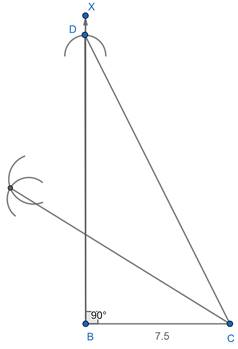Step 5: Let Y intersect BX at A.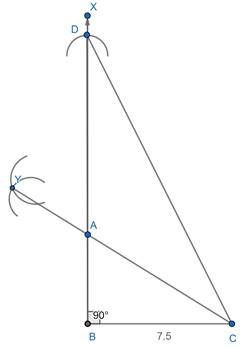Thus, ΔABC is the required triangle.Question 5.Construct a segment of a circle on a chord of length 5cm. containing the following angles.90°Answer:Given an angle of 90° and chord 5cmSteps of construction:Rough image: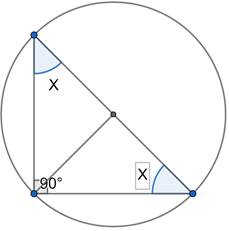Explanation:x + x + 90° = 180°[using sum of all angles in a triangle = 180°]⇒ 2x + 90° = 180°⇒ 2x = 180° - 90°⇒ 2x = 900°⇒ x =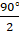= 45°Step 1: Draw a line segment AB = 5cm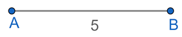Step 2: Draw an angle of 45° on point A and B to intersect at O.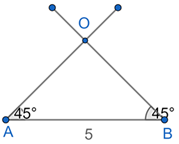Step 3: With centre ‘O’ and radius OA and OB, draw the circle.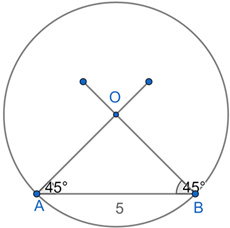Step 4: Mark a point ‘C’ on the arc of the circle. Join AC and BC.we get ∠CAB = 90°.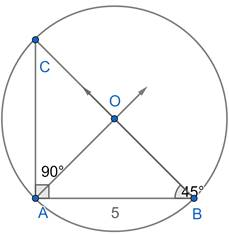Thus, ACB is the required circle segment.Question 6.Construct a segment of a circle on a chord of length 5cm. containing the following angles.45°Answer:Given an angle of 45° and chord 5cmSteps of construction:Step 1: Draw a line segment AB = 5cm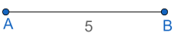Step 2: Draw an angle of 45° on point A and B to intersect at O.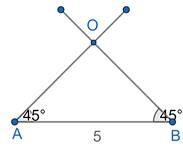Step 3: With centre ‘O’ and radius OA and OB, draw the circle.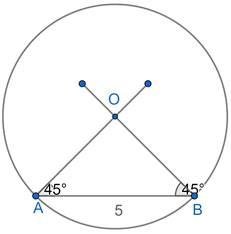Step 4: Mark a point ‘C’ on the arc of the circle. Join AC and BC.we get ∠ACB = 45°.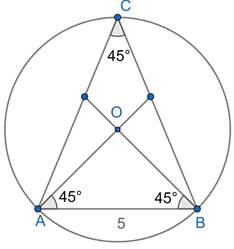Thus, ACB is the required circle segment.Question 7.Construct a segment of a circle on a chord of length 5cm. containing the following angles.120°Answer:Given an angle of 120° and chord 5cmRough Image :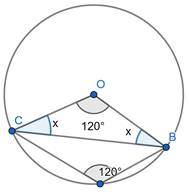Explanation:x + x + 120° = 180°[using sum of all angles in a triangle = 180°]⇒ 2x + 120° = 180°⇒ 2x = 180° - 120°⇒ 2x = 60°⇒ x =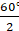= 30°Steps of construction:Step 1: Draw a line segment AB = 5cm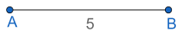Step 2: Draw an angle of 30° on point A and B to intersect at O.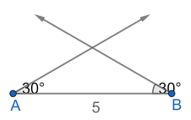Step 3: With centre ‘O’ and radius OA and OB, draw the circle.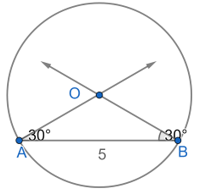Step 4: Mark a point ‘C’ under the chord AB and on the arc of thecircle . Join AC and BC.we get M∠A0B = 240°.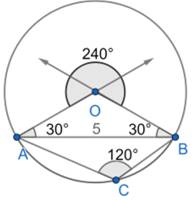Thus, ACB is the required circle segment.

PDF FILE TO YOUR EMAIL IMMEDIATELY PURCHASE NOTES & PAPER SOLUTION. @ Rs. 50/- each (GST extra)

HINDI ENTIRE PAPER SOLUTION

MARATHI PAPER SOLUTION

SSC MATHS I PAPER SOLUTION

SSC MATHS II PAPER SOLUTION

SSC SCIENCE I PAPER SOLUTION

SSC SCIENCE II PAPER SOLUTION

SSC ENGLISH PAPER SOLUTION

SSC & HSC ENGLISH WRITING SKILL

HSC ACCOUNTS NOTES

HSC OCM NOTES

HSC ECONOMICS NOTES

HSC SECRETARIAL PRACTICE NOTES

# 2019 Board Paper Solution

HSC ENGLISH SET A 2019 21st February, 2019

HSC ENGLISH SET B 2019 21st February, 2019

HSC ENGLISH SET C 2019 21st February, 2019

HSC ENGLISH SET D 2019 21st February, 2019

SECRETARIAL PRACTICE (S.P) 2019 25th February, 2019

HSC XII PHYSICS 2019 25th February, 2019

CHEMISTRY XII HSC SOLUTION 27th, February, 2019

OCM PAPER SOLUTION 2019 27th, February, 2019

HSC MATHS PAPER SOLUTION COMMERCE, 2nd March, 2019

HSC MATHS PAPER SOLUTION SCIENCE 2nd, March, 2019

SSC ENGLISH STD 10 5TH MARCH, 2019.

HSC XII ACCOUNTS 2019 6th March, 2019

HSC XII BIOLOGY 2019 6TH March, 2019

HSC XII ECONOMICS 9Th March 2019

SSC Maths I March 2019 Solution 10th Standard11th, March, 2019

SSC MATHS II MARCH 2019 SOLUTION 10TH STD.13th March, 2019

SSC SCIENCE I MARCH 2019 SOLUTION 10TH STD. 15th March, 2019.

SSC SCIENCE II MARCH 2019 SOLUTION 10TH STD. 18th March, 2019.

SSC SOCIAL SCIENCE I MARCH 2019 SOLUTION20th March, 2019

SSC SOCIAL SCIENCE II MARCH 2019 SOLUTION, 22nd March, 2019

XII CBSE - BOARD - MARCH - 2019 ENGLISH - QP + SOLUTIONS, 2nd March, 2019

# HSCMaharashtraBoardPapers2020

(Std 12th English Medium)

HSC ECONOMICS MARCH 2020

HSC OCM MARCH 2020

HSC ACCOUNTS MARCH 2020

HSC S.P. MARCH 2020

HSC ENGLISH MARCH 2020

HSC HINDI MARCH 2020

HSC MARATHI MARCH 2020

HSC MATHS MARCH 2020

# SSCMaharashtraBoardPapers2020

(Std 10th English Medium)

English MARCH 2020

HindI MARCH 2020

Hindi (Composite) MARCH 2020

Marathi MARCH 2020

Mathematics (Paper 1) MARCH 2020

Mathematics (Paper 2) MARCH 2020

Sanskrit MARCH 2020

Sanskrit (Composite) MARCH 2020

Science (Paper 1) MARCH 2020

Science (Paper 2)

Geography Model Set 1 2020-2021

MUST REMEMBER THINGS on the day of Exam

Are you prepared? for English Grammar in Board Exam.

Paper Presentation In Board Exam

How to Score Good Marks in SSC Board Exams

Tips To Score More Than 90% Marks In 12th Board Exam

How to write English exams?

How to prepare for board exam when less time is left

How to memorise what you learn for board exam

No. 1 Simple Hack, you can try out, in preparing for Board Exam

How to Study for CBSE Class 10 Board Exams Subject Wise Tips?

JEE Main 2020 Registration Process – Exam Pattern & Important Dates

NEET UG 2020 Registration Process Exam Pattern & Important Dates

How can One Prepare for two Competitive Exams at the same time?

8 Proven Tips to Handle Anxiety before Exams!